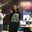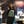Trusted answers to developer questions
Trusted Answers to Developer Questions

Related Tags

# How to convert CFG to NPDAAyesha Kanwal

Grokking Modern System Design Interview for Engineers & Managers

Ace your System Design Interview and take your career to the next level. Learn to handle the design of applications like Netflix, Quora, Facebook, Uber, and many more in a 45-min interview. Learn the RESHADED framework for architecting web-scale applications by determining requirements, constraints, and assumptions before diving into a step-by-step design process.

### Overview

A pushdown automaton (PDA) is a finite automaton with a stack for memory purposes. A nondeterministic pushdown automaton (NPDA) has one, or more than one, transition for each input that it reads.

A context-free grammar (CFG) is the generator of a language, whereas a PDA and NPDA are the recognizers of a non-regular language.

### Components of an NPDA

A PDA is defined by six tuples $$ where:

• $Q$ is the set of all the states
• $\sum$ is the language or the input alphabet
• $Γ$ is the stack alphabet
• $δ$ is the transition function and$δ : Q \times \sum_\epsilon \times Γ_\epsilon \rightarrow P(Q \times Γ_\epsilon)$
• $q_0$ is the start state and $q_0 \in Q$
• $F$ is the final state and $F \subseteq Q$

### Steps to convert CFG to NPDA

To convert a CFG to an NPDA, we follow these steps:

1. The stack is initially empty. Make the first transition as $\epsilon , \epsilon \rightarrow Γ$.
2. Before reading or popping the CFG, push the start variable on the stack.
3. Read nothing from the CFG in case of the start variable, pop it from the top of the stack, and push all the productions that the start variable produces. In the same step, read all the inputs of the CFG, pop, and push from the top of the stack according to the grammar rules mentioned in the CFG. This step transitions into a self-loop until all productions are read, popped, and pushed according to the CFG.
4. When there are no more productions to be read as input, pop the $Γ$symbol and push nothing. Make the last state the final state.

### Examples

The components of making a PDA are writing the steps that construct the PDA and the pushdown automaton itself.

Let's take an example of the following CFG:

#### Step 1: Push $\$ as the stack symbol

Input nothing from the CFG, pop nothing from the stack as it is already empty, and push $\$ as the stack symbol.

#### Step 2: Push $S$

Push $S$ on top of the stack, as it is the start variable. Input and pop nothing from the CFG and the stack, respectively.

#### Stack view

Stack after pushing S.

#### NPDA view

PDA after pushing S.

#### Step 3: Self-loop state

Read all possible inputs from the CFG, pop the same input from the stack, and push the productions they produce accordingly.

In the case of the start variable, as it is already on the top of the stack initially, pop it and push all the productions it creates.

#### Stack view

Stack after reading, popping, and pushing the CFG productions.

#### NPDA view

The PDA after reading, popping, and pushing the CFG productions

#### Step 4: Pop $\$

After all the productions have been read, pushed, and popped, only the $\$ is present on the stack. Pop it and transition into the final state.

The stack after popping $#### NPDA view The PDA after popping$

This is the final form of the NPDA formed by the CFG, where:

Note: To learn more about PDA, we can visit here.

RELATED TAGS

CONTRIBUTORAyesha Kanwal